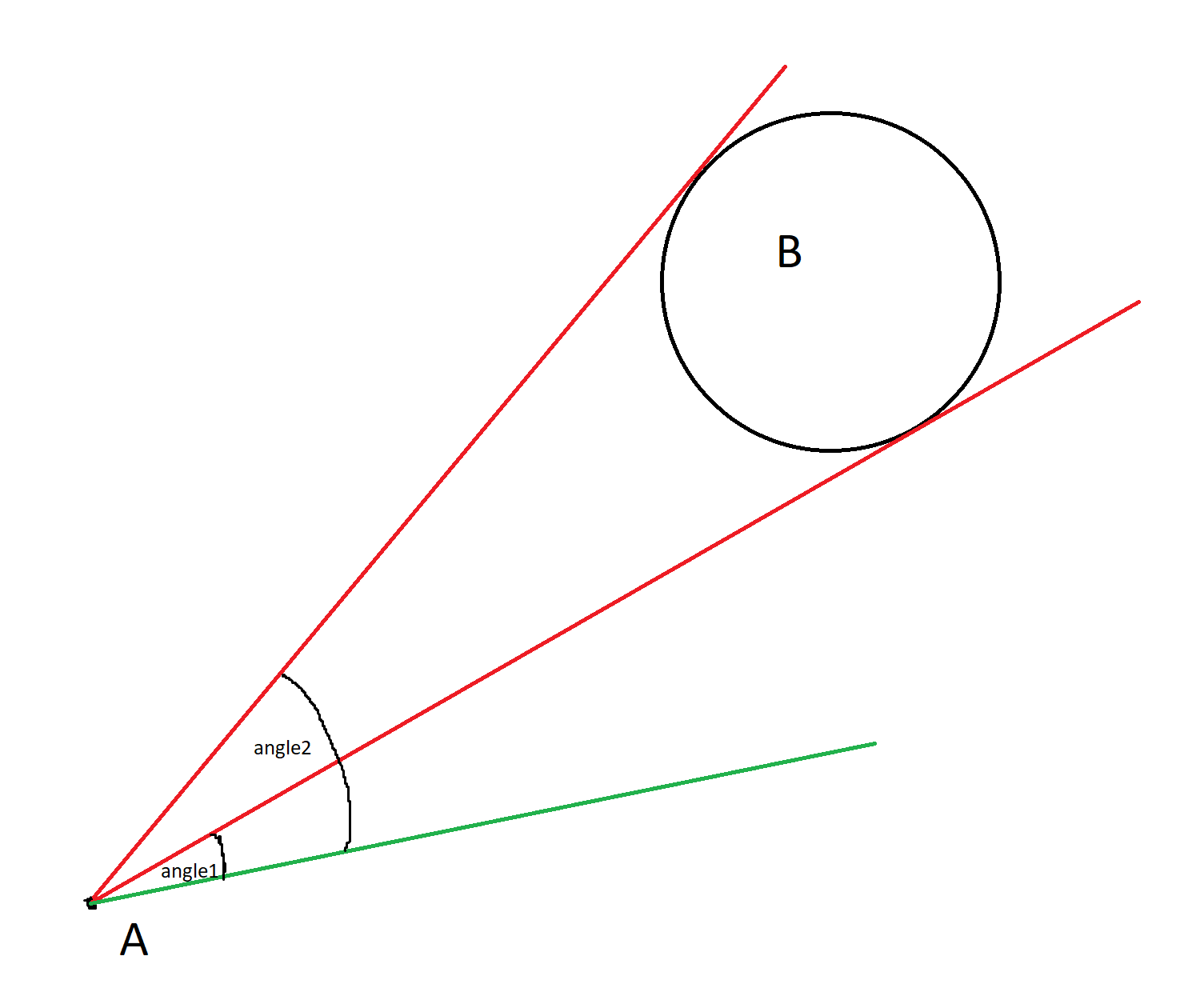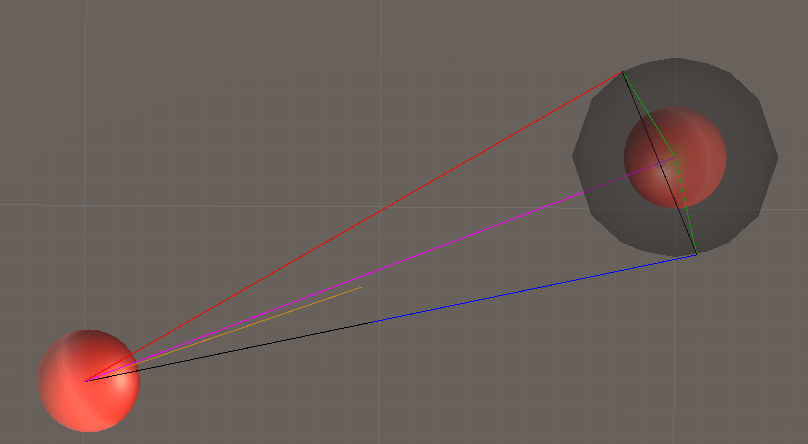# #StackBounty: #unity #2d #mathematics #movement #performance Improve velocity obstacle calculation algorithm/performance

### Bounty: 50

My goal

My goal is to calculate the (velocity) obstacle that is imposed by unit B onto unit A.
So I want to calculate the velocities from the center of unit A (circle) that will lead to a collision with unit B assuming unit B is not moving. The illegal velocities would be all velocities that are between the two tangents from the center of unit A to unit B (circle).
Thus I actually only need the angle of the two tangents relative to some other velocity.

Here is a simple drawing to illustrate my approach:The “other vector” (=green) is the “optimal velocity” for unit A, that is calculated through higher level pathfinding. My goal is then to choose the velocity that is closest to the optimal velocity, but not within the blocked/illegal velocities.

My approach

I already have a working demo, but it is too slow.

Note: In my implementation I extruded the radius of unit B by the radius of unit A.

My (primitive) approach to calculating the angles is:
1. Calculate the line from the center of unit A to Unit B
2. Calculate the first tangent point using trigonometry
3. Calculate the closest point from the first tangent point to the line from (1) (i call it the mirrorPoint)
4. Mirror the first tangent point along the mirrorPoint
5. Calculate angle1 and angle2 using Vector.SignedAngle

After wards i still have to do some work to find the closest legal vector to the optimal velocity, but that is not too time consuming.

Here is an image taken from the editor:The purple line is (1)
The green lines are the lines from the center of Unit B to the tangent points.
The black line (in unit B) are the lines from the mirrorPoint to the tangent points
The blue and the red line are the lines from the center of unit A to the tangent points
The orange line is the optimal velocity
The black line (starting at the center of Unit A) is the legal velocity that is closest to optimal velocity

Here is the code that i use to calculate the angles:

``````public Vector2 GetDesiredSteering(VelocityObstacleSteeringInput input)
{
TestUnit unit = input.Unit;
List<TestUnit> neighbors = GetNeighbors(unit);
foreach (TestUnit neighbor in neighbors)
{
Vector2 tangent1;
Vector2 tangent2;
CalculateTangent(unit.Pos, unit.Scale.x, neighbor.Pos, neighbor.Scale.x, out tangent1, out tangent2);

Vector2 relativeTangent1 = tangent1 - unit.Pos;
Vector2 relativeTangent2 = tangent2 - unit.Pos;
float angle1 = Vector2.SignedAngle(relativeTangent1, input.OptimalVelocity);
float angle2 = Vector2.SignedAngle(relativeTangent2, input.OptimalVelocity);
Debug.Log("angle1: " + angle1 + " angle2: " + angle2);
}

//return dummy for this stripped down version
return Vector2.up;
}

bool CalculateTangent(Vector2 unitPos, float unitRadius, Vector2 otherPos, float otherRadius, out Vector2 tangentPoint1, out Vector2 tangentPoint2)
{
Vector2 hypo = unitPos - otherPos;
float hypoLen = hypo.magnitude;
float ratio = combinedRadius / hypoLen;
float alpha = Mathf.Acos(ratio);
hypo.Normalize();
Vector2 centerToTangent = rotated * combinedRadius;
tangentPoint1 = otherPos + centerToTangent;
tangentPoint2 = GeometryHelper.CenterToOtherTangentPoint(otherPos, hypo, tangentPoint1, out Vector2 curMirrorPoint);
return true;
}

{
return new Vector2(
aPoint.x * c - aPoint.y * s,
aPoint.y * c + aPoint.x * s);
}

//linePointhas to be center of unit
public static Vector2 CenterToOtherTangentPoint(Vector2 linePoint, Vector2 lineDirection, Vector2 tangentPoint1, out Vector2 returnMirrorPoint)
{
Vector2 mirrorPoint = FindNearestPointOnLine(linePoint, lineDirection, tangentPoint1);
returnMirrorPoint = mirrorPoint;
Vector2 tangentPointToMirrorPoint = mirrorPoint - tangentPoint1;
return mirrorPoint + tangentPointToMirrorPoint;
}

public static Vector2 FindNearestPointOnLine(Vector2 origin, Vector2 direction, Vector2 point)
{
direction.Normalize();
Vector2 lhs = point - origin;

//t = how far to move from the origin along the direction to reach the closest point
float t = Vector2.Dot(lhs, direction);
return origin + direction * t;
}
``````

My Problem

I need to be able to scale this approach to at the very minmum 100 units (but I would very much prefer 200 units), which currently is not possible, because the algorithm/implementation is too slow.

So I am wondering if somebody can think of an improvement to this approach. Either mathematically(i.e. some better method/shortcut to calculate the angles) or other tips how to improve my code.

Get this bounty!!!

This site uses Akismet to reduce spam. Learn how your comment data is processed.